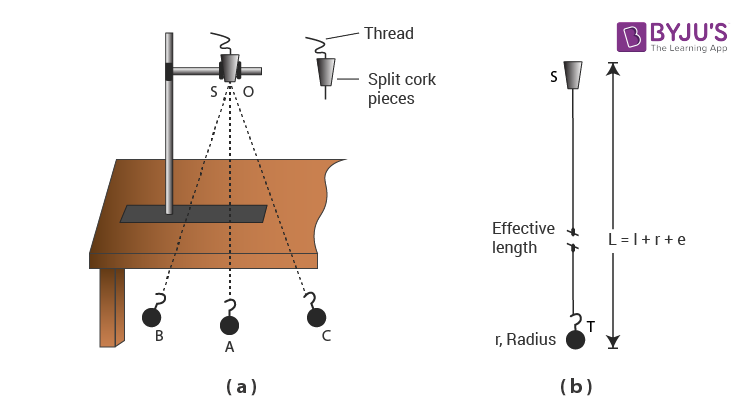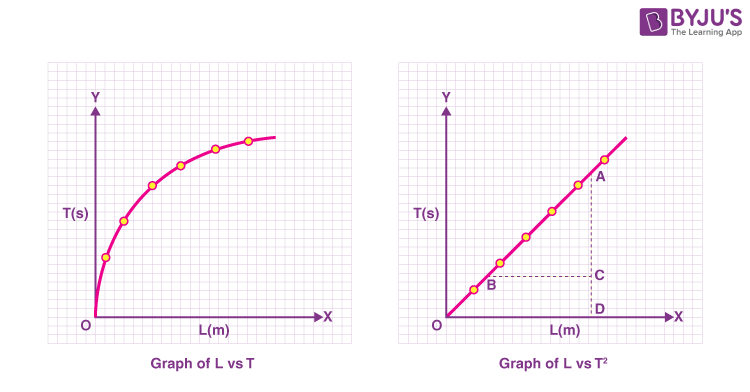# Using a simple pendulum, plot L-T and L-T2 graphs and use it to find the effective length of a second’s pendulum using appropriate graph

## Aim

Using a simple pendulum, plot L-T and L-T2 graphs and use it to find the effective length of the second’s pendulum.

## Apparatus and Material Required

• Clamp stand
• Split Cork
• Heavy metallic spherical bob with a hook
• Long and strong cotton thread
• Stopwatch
• Meter scale
• Graph Paper
• Pencil Eraser

## Principle

The simple pendulum exhibits Simple Harmonic Motion (SHM) as the acceleration of the pendulum bob is directly proportional to the displacement from the mean position and is always directed towards it. The time period (T) of a simple pendulum for oscillations of small amplitude is given by the relation

$$\begin{array}{l}T=2\pi\sqrt{L/G}\end{array}$$

Where L is the length of the pendulum and g is the acceleration of gravity

## Procedure

1. Place the clamp stand on the table. Tie the hook attached to the pendulum bob, to one end of the string of about 150 cm in length and the other end of the string through two half-pieces of a split cork.
2. Clamp the split cork firmly to the clamp stand such that the line of separation between the two pieces of the split cork is at right angles to the line OA along which the pendulum oscillates as given in the figure. Mark the edge of the table a vertical line parallel to and just behind the vertical thread OA, the position of the bob at rest. Take care that the bob hangs vertically (about 2 cm above the floor) beyond the edge of the table so that it is free to oscillate.
3. Measure the effective length of the simple pendulum as shown in the figure.1. Displace the bob not more than 15 degrees from the vertical position OA and then gently release it. If you notice the stand to be shaky, put a heavy object on its base. Make sure that the bob oscillates in a vertical plane about its rest and does not (i) spin about its own axis (ii) move up and down while oscillating (iii)revolve in an elliptic path around its mean position.
2. Keep the pendulum oscillating for a few minutes. After the completion of few oscillations, start the stopwatch as the thread attached to the bob crosses the mean position. Consider it as a zero oscillation.
3. Keep counting the oscillation 1,2,3…n every time the bob crosses the mean position. Stop the stopwatch at the count of n oscillations. For better results, n should be chosen such that the time taken to complete n oscillations is 50 s or more. Read the total time taken for n oscillations. Repeat the observation a few times by noting down the time for the same n number of oscillations. Once noted down, take the mean of the readings. Calculate the time for one oscillation, i.e., the time period T ( = t/n) of the pendulum.
4. Change the length of the pendulum, by about 10 cm. Repeat step 6 again for finding the time (t) for about 20 oscillations or more for the new length and find the mean time period. Take 5 or 6 more observations for different lengths of the pendulum and find the mean time period in each case.
5. Report observations in tabular form with proper units and significant figures.
6. Take effective length L along the x-axis and T2 (or T) along the y-axis, using the observed values from the table. Choose suitable scales on these axes to represent L and T2 (or T). Plot a graph between L and T2 as shown in figure 2 and also between L and T as shown in figure 1.

## Observation

The radius of the pendulum of the bob = ….. cm

Length of the hook = ….. cm

Least count of the meter scale = ….. mm

Least count of the stopwatch = ….. s

 S. No Length of the string from the top of the bob to the point of suspension l Effective length, L = (l+r+e) Number of oscillations counted, n Time for n oscillations t (s) Time period T (= t/n) cm m (i) (ii) (iii) Mean t (s)

## Plotting Graph

(i) L vs T Graph

Plot a graph between L versus T from observations recorded in the table above, taking L along x-axis and T along the y-axis. You will find that this graph is a curve, which is part of a parabola as shown in Figure 1.

(ii) L vs T2 Graph

Plot a graph between L versus T2 from observations recorded in the table, taking L along the x-axis and T2 along the y-axis. You will find that the graph is a straight line passing through the origin as shown in figure 2.

(iii) From the L versus T2 graph, determine the effective length of the second’s pendulum for T2 = 4s2.## Result

The graph L versus T is curved, convex upwards.

The graph L versus T2 is a straight line.

The effective length of the second’s pendulum from the L versus T2 graph is … cm.

## Viva Voice

Define simple pendulum.

Answer: A pendulum is defined as a single isolated particle suspended by a weightless Flexible and inextensible string with frictionless support.

Why is the word ‘simple’ used in simple pendulum?

Answer: Because the simple pendulum consists of mass m hanging from a string of length l and fixed at a pivot point P. The pendulums used in the wall clocks are known as a compound pendulum, and they have a metallic string instead of a thread.

Define simple harmonic motion (S.H.M).

Answer: A motion is called simple harmonic motion when:

(i)the magnitude of its acceleration is directly proportional to the displacement x from the mean position.
(ii) the direction of the acceleration is always towards the mean position.

What is the relationship between frequency and time period?

Answer: The relationship between frequency and time period is given by f = 1/T

What is restoring force?

Answer: The force that brings a vibrating body towards the mean position is known as the restoring force.

Can we use a cricket ball in the place of the bob?

Answer: No, we cannot. The bob must be as small as possible.